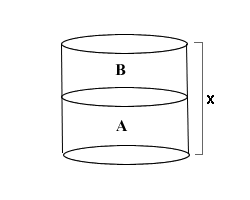Jan '09
23
MCAT Question of the Day | MCAT Course

• ### Q:

Suppose that you have an opened cylinder filled to the top with two equal quantities of fluids. Fluid A has a density of a and Fluid B has a density of b. If the height of the cylinder is x, what is the pressure of the fluid system at the bottom of the cylinder (assume that atmospheric pressure is Patm and that gravity is g)?### A

(bgx)/2 + (agx)/2

### B

(bgx)/2 + agx

### C

Patm + (bgx)/2 + agx

### D

Patm + (bgx)/2 + (agx)/2

Tags: Forces | Fluids | Suggest a tag
Subscribe

Connect

Questions? We're here to help!